# Introduction

To date, distribution and ecological niche models of marine species have generally only considered distributions in two-dimensional space, not accounting for depth. This may lead to mis-estimation of species distributions and subsequent diversity estimates, especially among pelagic and benthic species. Here, we present a possible way forward: generating species distribution models based on environmental data extracted at the depths where individuals were observed, and calibrated with three-dimensional sampling of pseudo-absences. We also provide some methods to project these models back into three-dimensional space and visualize the results.

Here are the packages you will need.

library(voluModel) # Because of course
library(dplyr) # To filter data
library(ggplot2) # For fancy plotting
library(rgdal,
options("rgdal_show_exportToProj4_warnings"="none")) # For vector stuff. Will eventually be replaced with sf.
library(raster) # For raster stuff. Will eventually be replaced with terra.
library(terra) # Now being transitioned in
library(sf) # Now being tranistioned in
library(lattice) # For plotting

# Data Inputs

First thing’s first. Distributional models are based on coordinates of species’ occurrences. We start with and example occurrence dataset of Steindachneria argentea, Luminous Hake, using data downloaded via R (R Core Team, 2020) from GBIF (Chamberlain et al., 2021; Chamberlain and Boettiger, 2017) and OBIS (Provoost and Bosch, 2019) via occCite (Owens et al., 2021). Here is how the occurrence data file is structured, and where the occurrences we will be using are located.

occs <- read.csv(system.file("extdata/Steindachneria_argentea.csv",
package='voluModel'))

# Filter points
occurrences <- occs %>%
dplyr::select(decimalLongitude, decimalLatitude, depth) %>%
dplyr::distinct() %>%
dplyr::filter(dplyr::between(depth, 1, 2000))

land <- rnaturalearth::ne_countries(scale = "small", returnclass = "sf")
pointMap(occs = occurrences, ptCol = "orange", landCol = "black",
spName = "Steindachneria argentea", ptSize = 3,
land = land)
##   decimalLongitude decimalLatitude  depth
## 1        -54.90000        7.450000 260.50
## 2        -54.08333        7.666667 450.00
## 3        -54.08333        7.666667 450.00
## 4        -56.87500        7.741667 365.76
## 5        -80.43333        9.300000  82.00
## 6        -59.78330        9.683300 274.00

Next, we load two environmental datasets from the World Ocean Atlas (Garcia et al., 2019): temperature (Locarnini et al., 2018) and apparent oxygen utilization (AOU; Garcia et al., 2019). I have chosen these variables for simplified illustrative purposes–we recommend you explore additional variables from the World Ocean Atlas and other sources. These data are supplied by the World Ocean Atlas as point shapefiles; the version supplied here has been cropped between -110 and -40 longitude and between -5 and 50 latitude to make it more space-efficient.

# Temperature
td <- tempdir()
unzip(system.file("extdata/woa18_decav_t00mn01_cropped.zip",
package = "voluModel"),
exdir = paste0(td, "/temperature"), junkpaths = T)
temperature <- readOGR(dsn = paste0(td, "/temperature"),
layer ="woa18_decav_t00mn01_cropped")
## OGR data source with driver: ESRI Shapefile
## Source: "/private/var/folders/xn/_tk36pd5517g_xxb5t9gh2qr0000gn/T/Rtmpm11zxP/temperature", layer: "woa18_decav_t00mn01_cropped"
## with 1633 features
## It has 102 fields
unlink(paste0(td, "/temperature"), recursive = T)

temperature@data[temperature@data == -999.999] <- NA

# Creating a bottom raster
temperatureBottom <- bottomRaster(temperature)

# Creating a RasterBrick
temperature <- rasterFromXYZ(cbind(temperature@coords,
temperature@data))

# Get names of depths
envtNames <- gsub("[d,M]", "", names(temperature))
envtNames[] <- "0"
names(temperature) <- envtNames

Let’s see what the top and bottom temperature files look like.

# How do these files look?
p1 <- oneRasterPlot(temperature[], land = land, landCol = "black",
title= "Temperature (C)")

p2 <- oneRasterPlot(temperatureBottom,land = land, landCol = "black",
title = "Temperature (C)")

temp <- c("Surface" = p1, "Bottom" = p2)
update(temp, strip  = strip.custom(strip.levels = TRUE,
horizontal = TRUE,
bg = "black",
fg = "white",
par.strip.text = list(col = "white", cex = 1.2, font = 2)))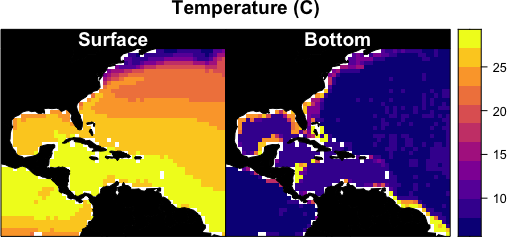The AOU dataset has been interpolated and smoothed using a thin plate spline algorithm. For more details on how to process environmental data, see the raster data tutorial. First, we load the data.

# Oxygen processing, pre-baked to save time
package='voluModel'))
package = 'voluModel'))
names(oxygenSmooth) <- names(temperature)

Then we plot it.

p3 <- oneRasterPlot(oxygenSmooth[], land = land, landCol = "black",
title= "Apparent Oxygen Utilization (µmol/kg),\n interpolated and smoothed")
p4 <- oneRasterPlot(oxygenBottom, land = land, landCol = "black",
title = "Apparent Oxygen Utilization (µmol/kg), interpolated and smoothed")
temp <- c("Surface" = p3, "Bottom" = p4)
update(temp, strip  = strip.custom(strip.levels = TRUE,
horizontal = TRUE,
bg = "black",
fg = "white",
par.strip.text = list(col = "white", cex = 1.2, font = 2)))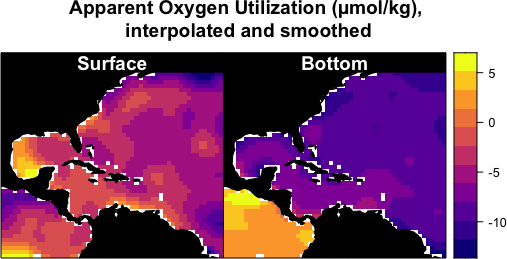Finally, we get data layer indices for each occurrence point by matching their depth of sampling to the appropriate environmental layer name.

# Gets the layer index for each occurrence by matching to depth
layerNames <- as.numeric(gsub("[X]", "", names(temperature)))
occurrences$index <- unlist(lapply(occurrences$depth, FUN = function(x) which.min(abs(layerNames - x))))
indices <- unique(occurrences$index) downsampledOccs <- data.frame() for(i in indices){ tempPoints <- occurrences[occurrences$index==i,]
tempPoints <- downsample(tempPoints, temperature[], verbose = FALSE)
tempPoints$depth <- rep(layerNames[[i]], times = nrow(tempPoints)) downsampledOccs <- rbind(downsampledOccs, tempPoints) } occurrences <- occurrences[,c("decimalLatitude", "decimalLongitude", "depth")] rm(indices, layerNames, tempPoints, i, downsampledOccs, occs) # A point about 3D environmental data extraction At this point, we can demonstrate one of the key contributions of voluModel– an improvement in how the environmental data are extracted at species’ occurrences. voluModel samples environmental conditions at the depth where each record was located, instead of extrapolating to surface or bottom conditions. How important is this? First, let’s look at temperature.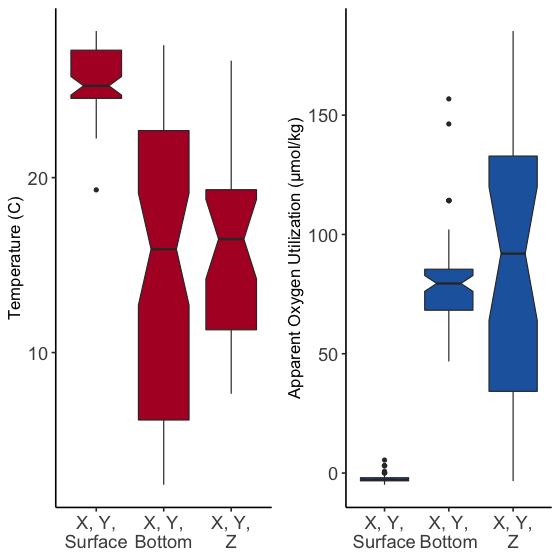Temperature extracted at the appropriate depth (3D) is significantly lower than temperature extracted at the surface. The mean value of temperature is similar between the bottom and the 3D extractions, but the range of 3D values is narrower than those extracted at the bottom. AOU based on 3D extraction is significantly higher than AOU extracted at the surface. The mean value of AOU is again similar between the bottom and the 3D extractions; the range of 3D values is broader than those extracted at the bottom, but contains no statistical outliers, i.e. greater than 1.5 * interquartile range from the hinge of the boxplot (black dots). # Data sampling Some modeling methods require the generation of a sampling region from which pseudoabsence or background points are drawn. For this introduction, we are showing you a simple envelope method that does not require background sampling. Instead, I will be applying the same principle to define the study region to which I project the envelope model, so we have a product that makes biological sense in terms of the question “Where are we most likely to encounter Lumious Hakes?” See the background sampling tutorial for more information on voluModel’s marineBackground() function, and the generalized linear model tutorial for an example of a modelling application that uses a background sampling region. studyRegion <- marineBackground(occurrences, buff = 1000000) landVect <- vect(land) landVect <- terra::project(landVect, y = studyRegion) plot(studyRegion, border = F, col = "gray", main = "Points and Background Sampling", axes = T) plot(landVect, col = "black", add = T) points(occurrences[,c("decimalLongitude", "decimalLatitude")], pch = 20, col = "red", cex = 1.5)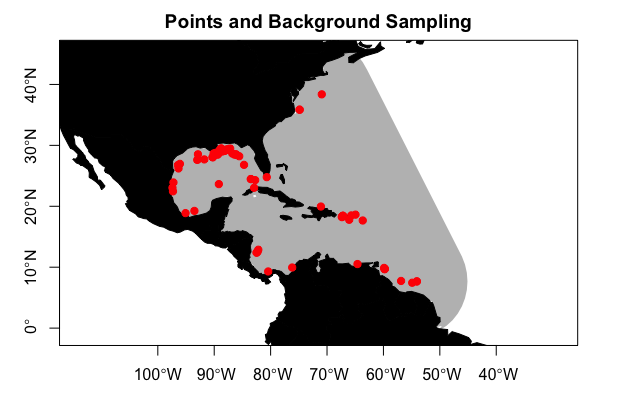For our simple niche envelope models, we need to draw the environmental variable data from each occurrence voxel. For comparison with previous modeling approaches, I will extract data from the surface, from the bottom, and from the 3D coordinate for each variable. # Surface Presences oxyVals <- extract(x = oxygenSmooth[], occurrences[,c("decimalLongitude", "decimalLatitude")]) tempVals <- extract(x = temperature[], occurrences[,c("decimalLongitude", "decimalLatitude")]) vals <- cbind(occurrences, oxyVals, tempVals) colnames(vals) <- c("decimalLongitude", "decimalLatitude", "depth", "AOU", "Temperature") vals <- vals[complete.cases(vals),] row.names(vals) <- NULL occsWdataSurface <- vals # Bottom Presences oxyVals <- extract(x = oxygenBottom, occurrences[,c("decimalLongitude", "decimalLatitude")]) tempVals <- extract(x = temperatureBottom, occurrences[,c("decimalLongitude", "decimalLatitude")]) vals <- cbind(occurrences, oxyVals, tempVals) colnames(vals) <- c("decimalLongitude", "decimalLatitude", "depth", "AOU", "Temperature") vals <- vals[complete.cases(vals),] row.names(vals) <- NULL occsWdataBottom <- vals # 3D Presences oxyVals <- xyzSample(occs = occurrences, oxygenSmooth) ## Using decimalLongitude, decimalLatitude, and depth ## as x, y, and z coordinates, respectively. tempVals <- xyzSample(occs = occurrences, temperature) ## Using decimalLongitude, decimalLatitude, and depth ## as x, y, and z coordinates, respectively. vals <- cbind(occurrences, oxyVals, tempVals) colnames(vals) <- c("decimalLongitude", "decimalLatitude", "depth", "AOU", "Temperature") vals <- vals[complete.cases(vals),] row.names(vals) <- NULL occsWdata3D <- vals rm(oxyVals, tempVals, vals) # Niche envelope models Now I will demonstrate how to construct simple 2D envelope models, in which we define suitable conditions as those within the maximum and minimum conditions at which Steindachneria argentea has been observed. The results will be simple 2D distribution maps–one based on surface conditions and one based on bottom conditions. # Get limits, surface tempLims <- quantile(occsWdataSurface$Temperature,c(0, 1))
aouLims <- quantile(occsWdataSurface$AOU,c(0, 1)) # Reclassify environmental bricks to presence/absence, surface temperaturePresence <- reclassify(temperature[], rcl = c(-Inf,tempLims[],0, tempLims[], tempLims[], 1, tempLims[], Inf, 0)) AOUpresence <- reclassify(oxygenSmooth[], rcl = c(-Inf, aouLims[],0, aouLims[], aouLims[], 1, aouLims[], Inf, 0)) # Put it all together, surface envelopeModelSurface <- temperaturePresence * AOUpresence studyRegion <- as(studyRegion, "Spatial") envelopeModelSurface <- raster::mask(raster::crop(envelopeModelSurface, studyRegion), mask = studyRegion) # Get limits, bottom tempLims <- quantile(occsWdataBottom$Temperature,c(0, 1))
aouLims <- quantile(occsWdataBottom$AOU,c(0, 1)) # Reclassify environmental bricks to presence/absence, bottom temperaturePresence <- reclassify(temperatureBottom, rcl = c(-Inf,tempLims[],0, tempLims[], tempLims[], 1, tempLims[], Inf, 0)) AOUpresence <- reclassify(oxygenBottom, rcl = c(-Inf, aouLims[],0, aouLims[], aouLims[], 1, aouLims[], Inf, 0)) # Put it all together, bottom envelopeModelBottom <- temperaturePresence * AOUpresence envelopeModelBottom <- mask(crop(envelopeModelBottom, studyRegion), mask = studyRegion) How do these 2D models compare? voluModel has a function to conveniently generate a formatted comparison between two presence/absence distribution rasters rasterComp(). rasterComp(rast1 = envelopeModelSurface, rast2 = envelopeModelBottom, rast1Name = "Surface", rast2Name = "Bottom", land = land, landCol = "black", title = "Comparison between surface and bottom envelope models")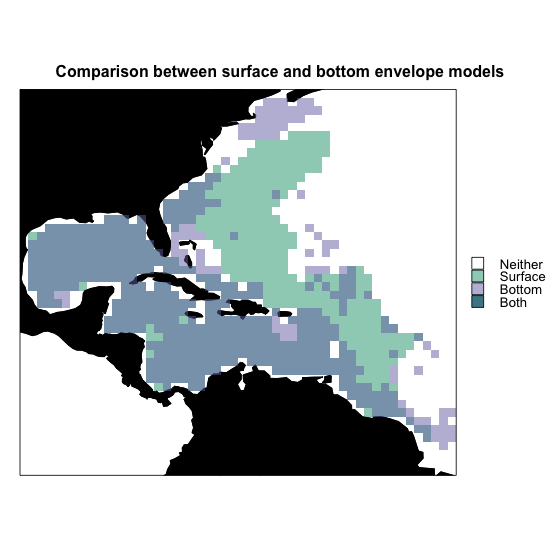Now I’ll demonstrate how to make a 3D envelope model. Generating the model is exactly the same–any conditions between the maximum and minimum values at which Luminous Hakes were observed are considered suitable. The estimates are, of course, based on 3D environmental data extractions. The other key difference is that the envelope model is projected to each layer in each of the raster bricks (essentially generating a single variable model for each variable). The single-variable models are then multiplied together to generate a full model. # Get limits tempLims <- quantile(occsWdata3D$Temperature,c(0, 1))
aouLims <- quantile(occsWdata3D$AOU,c(0, 1)) # Reclassify environmental bricks to presence/absence temperaturePresence <- reclassify(temperature, rcl = c(-Inf,tempLims[],0, tempLims[], tempLims[], 1, tempLims[], Inf, 0)) AOUpresence <- reclassify(oxygenSmooth, rcl = c(-Inf, aouLims[],0, aouLims[], aouLims[], 1, aouLims[], Inf, 0)) # Put it all together envelopeModel3D <- temperaturePresence * AOUpresence envelopeModel3D <- mask(crop(envelopeModel3D, studyRegion), mask = studyRegion) names(envelopeModel3D) <- names(temperature) How does the projection look? plotLayers plots a transparent layer of suitable habitat for each depth layer. The redder the color, the shallower the layer, the bluer, the deeper. The more saturated the color, the more layers with suitable habitat. We will only look at projections within the study area and at depths the species was observed. # Get indices of model-relevant layers layerNames <- as.numeric(gsub("[X]", "", names(envelopeModel3D))) occurrences$index <- unlist(lapply(occurrences$depth, FUN = function(x) which.min(abs(layerNames - x)))) indices <- sort(unique(occurrences$index))

plotLayers(envelopeModel3D[[min(indices):max(indices)]],
title = "Envelope Model of Luminous Hake,\n 20 to 700m",
land = land)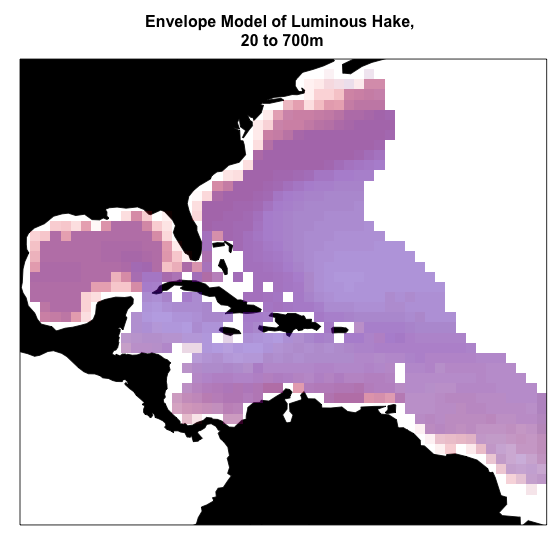Interestingly, the bottom model did not predict the suitable habitat recovered by the surface model in the Sargasso Sea. The 3D model predicts suitable habitat in the Sargasso Sea, but only at lower depths.

Of course, this is just the introduction. Please explore the other vignettes for more features and workflow examples. We welcome submissions from the community for other types of modeling algorithms, functions, and/or features you would like to see. Submit your suggestions as issues here.